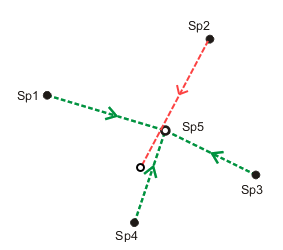# Measurements and accuracy

## What is a measurement?

A measurement is an observed numerical value that is an estimate of the true size of a quantity. All measurements are estimates of the true size and thus all measurements will have some degree of uncertainty.

Uncertainty in measurements can be best explained by considering the following example: Several people are asked to calculate the volume of water in a fish tank using a measuring tape. To do this, they must measure the length and breadth of the tank and the depth of the water. They are asked to measure as accurately as possible and to estimate fractions of the measurement unit of the measurement tape. Each person will derive a slightly different volume based on their estimates, and most calculated volumes will be close to the true value. Some calculations, however, may be incorrect due to mistakes in one or more of the measurements.

Uncertainty in measurements exists for the following reasons:

• The observer makes estimates.
• There is imperfection in the measuring equipment.
• The environment affects the measurement.
• The behavior of the equipment, observer, and environment cannot always be predicted.

### Measurement error versus measurement mistakes

Measurement uncertainty is known as measurement error, and all measurements contain some degree of error. Measurement mistakes are different from measurement error and occur when incorrect measurements are made. Measurement mistakes should be excluded from the calculation or estimation of a value.

When parcel boundaries are surveyed, they are susceptible to both measurement error and measurement mistakes. To get close to the true values of measured parcel boundaries, the following needs to be done:

• Identify and remove measurement mistakes.
• Apply mathematical and statistical methods such as a least-squares adjustment to account for measurement error and get as close to the true values as possible.

## What is measurement redundancy?

Random error in measurements can be detected and reduced by repeating measurements of the same quantity, for example, measuring the distance multiple times between two points. However, this is not sufficient to detect systematic error such as calibration errors with the measurement tape.

To detect and minimize both random and systematic errors, it is common practice to establish a network of measurements where each point is measured from a number of different points. Coordinates computed from measurements from different points can be compared to each other to detect errors. For a single point, any set of coordinates that differs significantly from the other coordinates computed by other measurements indicates an error and is known as an outlier.

The more measurement redundancy in the network, the better the chances are of detecting and controlling errors.## Measurement accuracy

Line dimensions and point coordinates are derived from survey measurements that have associated accuracies. Line dimensions and coordinates will therefore have associated accuracies as well. In general, the more recently the survey measurements were taken, the more accurate they are.

### Standard deviations

Standard deviation is the measure of the spread of values when repeatedly measuring the same target. For example, if a surveyor were to measure the same target point repeatedly with the same instrument, he or she would want the spread or range of values to be as close to each other as possible (in other words, the standard deviation should be as small as possible). Standard deviation is an indication of the accuracy of a measurement.

### Standard deviations of dimensions

In the parcel fabric, standard deviations are specified in the Direction Accuracy and Distance Accuracy fields for parcel line dimensions. In general, the standard deviations of dimensions are lower (higher accuracy) when the dimensions are from more recent records. When running a least squares analysis, if no standard deviations are provided, the defaults of 30 seconds for directions and 0.15 meters (0.59 feet) for distances are used.

The standard deviation values in the Direction Accuracy and Distance Accuracy fields act as weights on parcel lines in a least- squares adjustment. The lower the standard deviation values, the higher the accuracies are, and the higher the weights in the least squares adjustment. Dimensions with higher weights have more influence on the outcome of the least squares adjustment.

Standard deviations act as weights on parcel lines when running both a consistency check and a weighted least squares adjustment.

### Standard deviations of points

Standard deviations are specified for points when they are used as weighted control points in the least squares adjustment. Standard deviations are specified in the XY Accuracy field of the parcel fabric Points feature class. Standard deviations should only be specified for points when they are to act as weighted control points in a weighted least squares adjustment.

The lower the standard deviation value, the higher the accuracy of the point, and the higher its weight is in the adjustment. Points with higher weights constrain the adjustment more, have more of an influence on the outcome of the adjustment, and receive a smaller correction to their coordinates.